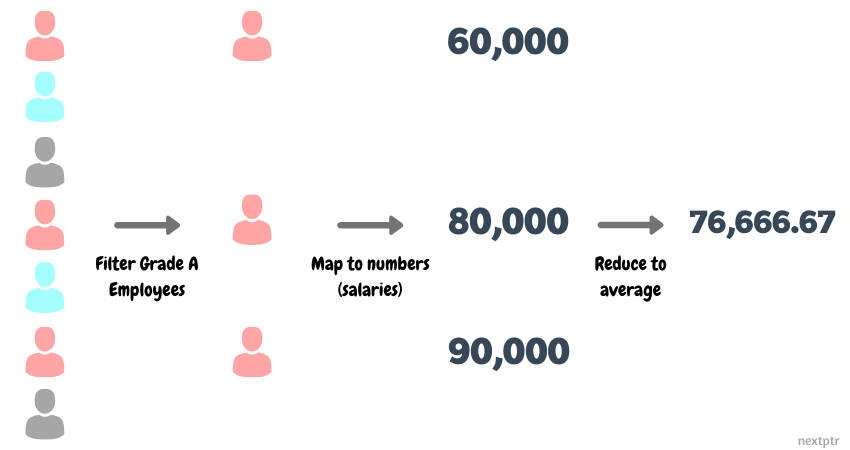# Introduction to Stream API through map-reduce

The Java 8 Stream API provides excellent support for the map-reduce functional programming model.

## Overview of Stream API

The Java 8 Stream API provides classes to perform functional-style operations in a pipeline on a stream of elements. Working on collections often requires looping over the items. The Stream API allows programmers to concentrate on only the necessary tasks, thus eliminating the need for writing the boilerplate loops. For instance, the following code calculates the sum of all even numbers in a list:

``````List<Integer> ints = Arrays.asList(2,3,2,6,5,7,8);

//Calculate sum of even numbers
int sum = ints.stream() //get stream
.filter((n) -> n%2 == 0) //filter even numbers
.mapToInt(Integer::intValue) //map to primitive int
.sum();
``````

At the core of the Stream API, are stream interfaces (e.g., Stream< T>, IntStream, DoubleStream), collectively known as the streams. The streams support various operations like map, filter, reduce, and collect, which can be chained together to form a pipeline. The stream operations can be either intermediate or terminal. A stream pipeline consists of a source (e.g., a collection, an array), zero or more intermediate operations (such as filter, map), and a terminal operation (such as sum, reduce, collect). Intermediate operations return a new stream of processed elements, and a terminal operation reduces or folds the elements to a result.

The stream operations can be performed in parallel (possibly) to boost the performance; however, this feature is outside the scope of this article. In the next two sections, we would explain how a stream pipeline is an epitome of the map-reduce paradigm, a framework for transforming and aggregating data in meaningful results.

## Map-Reduce with Stream API

Map-Reduce is a functional programming model for transforming and aggregating data. To understand map-reduce, let's consider a collection of Employee records fetched from a database:

``````// Employee record.
class Employee {
//Constructors...
String firstName;
String lastName;
int salary; //annual salary
char gender; //'M' or 'F'
String department; //e.g., "Sales"
//..more fields
}

List<Employee> employees = new ArrayList<>;
``````

Applications often have varied needs for data from a source (a collection of Employee objects in this case). Suppose we need to calculate the average salary of grade 'A' employees. We can solve this problem by writing a loop to calculate the sum of grade 'A' employees' salaries and then compute the average salary, as shown below:

``````int sumSalary = 0; //Sum
int count = 0; //Count of employees
for(Employee e : employees) {
sumSalary += e.salary;
count++;
}
}

//Calculate average salary
double avgSalary = count > 0 ? sumSalary/count : 0;
``````

However, in the map-reduce framework, this problem can be solved in three combined steps: first filter the grade 'A' employees, then map or transform the Employee objects to wages, and finally reduce or calculate the average of wages. The following image illustrates the concept:We can implement the above described map-reduce solution with the streams as follows:

``````OptionalDouble avgSalary = employees.stream()
.mapToInt((e) -> e.salary) //get IntStream of salaries
.average(); //get average
``````

Note how the functional map-reduce approach is different from our first solution with a loop. With map-reduce, we apply operations over data as opposed to the code over data. A map-reduce pipeline is analogous to a query on a data source.

The Stream API has various intermediate operations for filtering (e.g., filter, distinct, allMatch) and transforming (e.g., map, flatMap); and terminal operations for collection (e.g., collect) and reduction (e.g., reduce, sum, count, average). These are a few more examples of the map-reduce on the Employee collection:

Get a list of all employees from a specific department:

``````List<Employee> salesEmps = employees.stream()
.filter((e) -> e.department == "Sales") //Filter
.collect(Collectors.toList()); //Collect into a list
``````

Get a list of full names of all employees:

``````//Create a list of full names ("FirstName LastName")
List<String> names = employees.stream()
.map((e) -> e.firstName + " " + e.lastName) //map to full name
.collect(Collectors.toList()); //Collect into a list
``````

Get the maximum salary from a particular department:

``````//Max salary in Sales department
OptionalInt maxSalary = employees.stream()
.filter((e) -> e.department == "Sales") //filter
.mapToInt((e) -> e.salary) //map to int salaries
.max(); //get max salary
``````

Count the number of female employees:

``````long numFemales = employees.stream()
.filter((e) -> e.gender == 'F') //Filter females
.count(); //get count
``````

As we can see that map-reduce with the streams is great for readability and ease-of-implementation. Also, the streams are designed by keeping performance into consideration. For efficiency, the streams are designed as lazy, which means the intermediate stream operations in a pipeline are not evaluated until the terminal operation is executed.

## Conclusion

The Stream API is an excellent part of Java's repertoire of map-reduce like functional programming. By careful consideration, the streams can help reduce the boilerplate code related to data queries from standard collections.